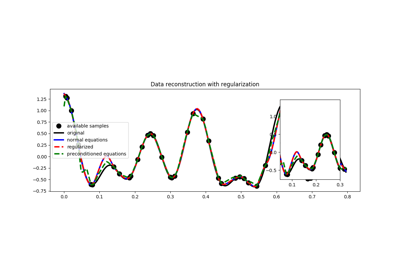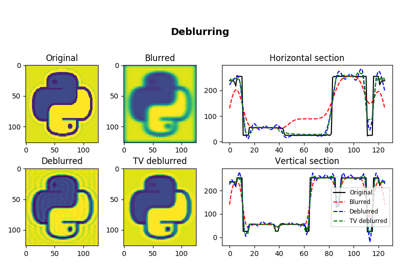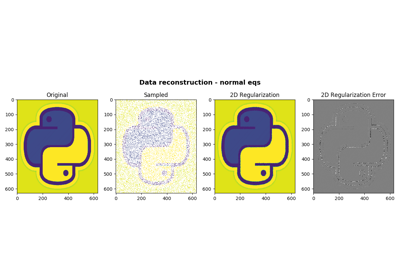# pylops.optimization.leastsquares.NormalEquationsInversion¶

pylops.optimization.leastsquares.NormalEquationsInversion(Op, Regs, data, Weight=None, dataregs=None, epsI=0, epsRs=None, x0=None, returninfo=False, **kwargs_cg)[source]

Inversion of normal equations.

Solve the regularized normal equations for a system of equations given the operator Op, a data weighting operator Weight and a list of regularization terms Regs

Parameters: Op : pylops.LinearOperator Operator to invert Regs : list Regularization operators (None to avoid adding regularization) data : numpy.ndarray Data Weight : pylops.LinearOperator, optional Weight operator dataregs : list, optional Regularization data (must have the same number of elements as Regs) epsI : float, optional Tikhonov damping epsRs : list, optional Regularization dampings (must have the same number of elements as Regs) x0 : numpy.ndarray, optional Initial guess returninfo : bool, optional Return info of CG solver **kwargs_cg Arbitrary keyword arguments for scipy.sparse.linalg.cg solver xinv : numpy.ndarray Inverted model. istop : int Convergence information: 0: successful exit >0: convergence to tolerance not achieved, number of iterations <0: illegal input or breakdown

RegularizedInversion
Regularized inversion
PreconditionedInversion
Preconditioned inversion

Notes

Solve the following normal equations for a system of regularized equations given the operator $$\mathbf{Op}$$, a data weighting operator $$\mathbf{W}$$, a list of regularization terms $$\mathbf{R_i}$$, the data $$\mathbf{d}$$ and regularization damping factors $$\epsilon_I$$ and $$\epsilon_{{R}_i}$$:

$( \mathbf{Op}^T \mathbf{W} \mathbf{Op} + \sum_i \epsilon_{{R}_i}^2 \mathbf{R}_i^T \mathbf{R}_i + \epsilon_I^2 \mathbf{I} ) \mathbf{x} = \mathbf{Op}^T \mathbf{W} \mathbf{d} + \sum_i \epsilon_{{R}_i}^2 \mathbf{R}_i^T \mathbf{d}_{R_i}$

## Examples using pylops.optimization.leastsquares.NormalEquationsInversion¶03. Solvers04. Image deblurring05. 2D Interpolation